Home > A2C > Chapter 9 > Lesson 9.1.1 > Problem9-14

9-14.
1. Solve the equations below. Homework Help ✎

1.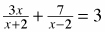2.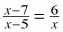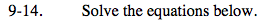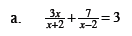Multiply both sides by (x + 2)(x − 2).

3x(x − 2) + 7(x + 2) = 3(x + 2)(x − 2)
3x² − 6x + 7x + 14 = 3(x2 − 4)
3x2 + x + 14 = 3x2 − 12

x = −26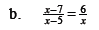x = 10, x = 3